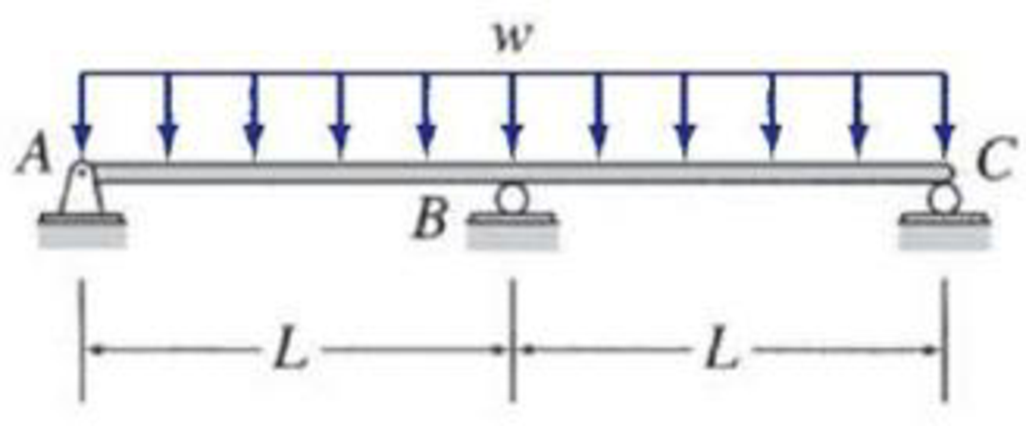# 13.9 through 13.12 Determine the reactions and draw the shear and bending moment diagrams for the beams shown in Figs. P13.9–P13.12 using the method of consistent deformations. Select the reaction at the interior support to be the redundant. FIG. P13.10, P13.31

#### Solutions

Chapter
Section
Chapter 13, Problem 10P
Textbook Problem
1 views

## 13.9 through 13.12 Determine the reactions and draw the shear and bending moment diagrams for the beams shown in Figs. P13.9–P13.12 using the method of consistent deformations. Select the reaction at the interior support to be the redundant.FIG. P13.10, P13.31

To determine

Calculate the support reactions for the given structure using method of consistent deformation.

Sketch the shear and bending moment diagrams for the given structure.

### Explanation of Solution

Given information:

The structure is given in the Figure.

Apply the sign conventions for calculating reactions, forces and moments using the three equations of equilibrium as shown below.

• For summation of forces along x-direction is equal to zero (Fx=0), consider the forces acting towards right side as positive (+) and the forces acting towards left side as negative ().
• For summation of forces along y-direction is equal to zero (Fy=0), consider the upward force as positive (+) and the downward force as negative ().
• For summation of moment about a point is equal to zero (Matapoint=0), consider the clockwise moment as positive when moment taken from left and the counter clockwise moment as positive when moment taken from right.

Calculation:

Consider the flexural rigidity EI of the beam is constant.

Sketch the free body diagram of the beam as shown in Figure 1.

Refer Figure 1,

Find the degree of indeterminacy of the structure:

Degree of determinacy of the beam is equal to the number of unknown reactions minus the number of equilibrium equations.

The beam is supported by 4 support reactions (r) and the number of equilibrium equations (e) is 3.

i=re=43=1

Therefore, the degree of indeterminacy of the beam is i=1.

Select the vertical reaction By at the supports B as redundant.

Release the support B and consider the downward deflection due to external load at B as ΔBO

Sketch the loading diagram with deflected shape as shown in Figure 2.

Refer Figure 2,

The formula for maximum central deflection of simply supported beam with uniformly distributed load as follows.

Δmax=5wL4384EI        (1)

Here, w is the uniformly distributed load through the beam, L is the total length of the beam, and EI is the flexural rigidity.

Find the downward deflection at B.

Substitute 2L for L in Equation (1).

ΔBO=5w(2L)4384EI=5wL424EI

Find the upward deflection at B.

Consider the upward deflection due to unit load at B as fBB

Sketch the beam with unit load at B with deflected shape due to unit load as shown in Figure 3.

Refer Figure 3,

The formula for maximum central deflection of simply supported beam with point load as follows.

Δmax=WL348EI        (2)

Here, W is the central point load through the beam, L is the total length of the beam, EI is the flexural rigidity.

Find the upward deflection at B.

Substitute 1 unit for W and 2L for L in Equation (1).

fBB=(1)(2L)348EI=L36EI

Find the vertical reaction at B using compatibility equation.

ΔBO+fBBBy=0

Substitute 5wL424EI for ΔBO and L36EI for fBB.

5wL424EI+(L36EI)By=0L36EIBy=5wL424EIBy=5wL4

Therefore, the vertical reaction at B is By=5wL4_.

Refer Figure 1,

Find the vertical reaction at A and C.

The loading is symmetric in the beam. Therefore, the reaction at A is equal to reaction at C (Ay=Cy)

### Still sussing out bartleby?

Check out a sample textbook solution.

See a sample solution

#### The Solution to Your Study Problems

Bartleby provides explanations to thousands of textbook problems written by our experts, many with advanced degrees!

Get Started

Find more solutions based on key concepts
Describe the process by which engineers evaluate alternatives.

Engineering Fundamentals: An Introduction to Engineering (MindTap Course List)

What steps might you be able to take if you are unsuccessful with your attempts to remove online information th...

Enhanced Discovering Computers 2017 (Shelly Cashman Series) (MindTap Course List)

How does the role of main memory differ from the role of secondary storage?

Principles of Information Systems (MindTap Course List)

If your motherboard supports ECC DDR3 memory, can you substitute non-ECC DDR3 memory?

A+ Guide to Hardware (Standalone Book) (MindTap Course List)